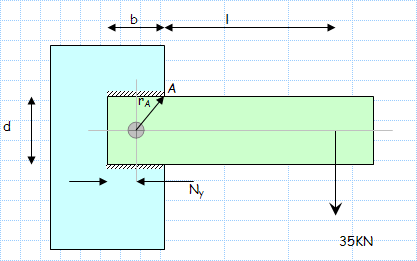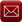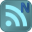You have downloaded this file 0 times in the last 24 hours, limit is 100.

## Weld line assessment - 2 horizontal welds.xls This location is for Registered Users Only.Perhaps you need to login or register.

Description:The method of assessing fillet welds groups treating welds as lines is reasonably safe and conservative and is very convenient to use.

a) Weld subject to bending....See table below for typical unit areas and unit Moments of Inertia

1) The area of the fillet weld Au..(unit thickness) is calculated assuming the weld is one unit thick..

2) The (unit) Moment of Inertia Iu is calculated assuming the weld is one unit thick..

3) The maximum shear stress due to bending is determined...

4) The maximum shear stress due to direct shear is determined..

5) The resultant stress

6) By comparing the design stress Ss with the resultant stress

a) Weld subject to torsion...See table below for typical unit areas and unit Polar moments of Inertia

A fillet weld subject to torsion is easily assessed as follows.

1) The area of the fillet weld Au (unit thickness) is calculated assuming the weld is one unit thick

2) The (unit) Polar Moment of Inertia Ju is calculated assuming the weld is one unit thick.. The polar moment of inertia J = Ixx + Iyy

3) The maximum shear stress due to torsion is determined...

4) The maximum shear stress due to direct shear is determined..

5) The resultant stress

6)By comparing the design stress Ss with the resultant stress

Calculation Reference
Schaum's Outline - Machine Design

Submitted By:
Submitted On:
29 Jan 2008
File Size:
58.50Kb
376
File Version:
1.0
Rating:Total Votes:5
harryburt 12 years ago
Looks like Blodgett's approach, which I have always found effective. I would like to see a metric/inch choice. (Unless I missed it. If so, I rate this a 5)
harryburt 12 years ago
Looks like Blodgett's approach, which I have always found effective. I would like to see a metric/inch choice. (Unless I missed it. If so, I rate this a 5)

We have 305 guests and 9 members onlineEmail (preferred method of contact)US +1 617 5008224EU +44 113 8152220